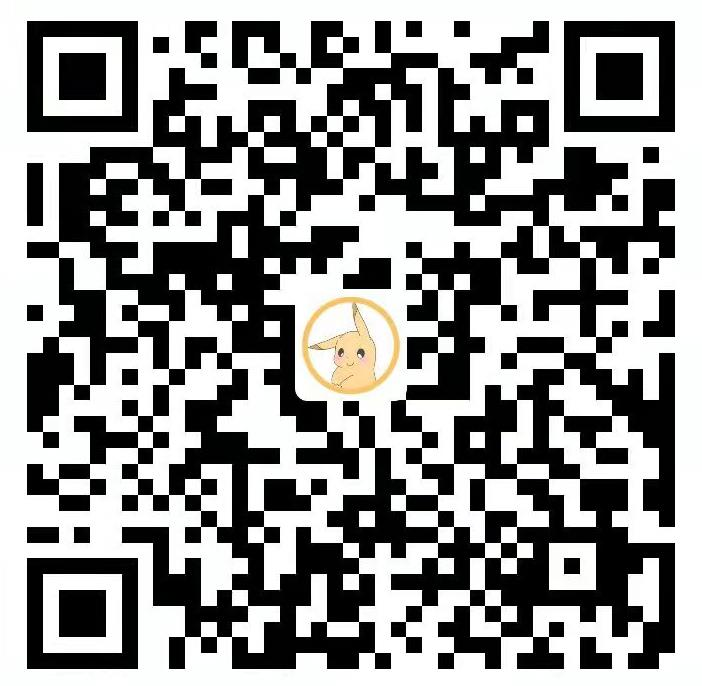## 题目描述

Sept 希望入场后可以看到所有名师的图画。当然，他想最小化购买门票的价格。

## 输入输出样例

12 5
2 5 3 1 3 2 4 1 1 5 4 3


2 7


## 说明/提示

#### 数据规模与约定

• 对于 30% 的数据，有 $n\le200，m\le20$
• 对于 60% 的数据，有 $n\le10^5，m\le10^3$
• 对于 100% 的数据，有 $1\leq n\le10^6，1 \leq a_i \leq m\le2\times10^3$

## 题目解析

1. 区间内不重复的元素个数有m个。
2. 满足条件1的前提下，区间要最小。

## 代码实现

#include <iostream>
#include <cstdio>
using namespace std;
const int N=1e6+5;
int a[N];
int n,m;
int v;//v[x]=画家x在区间内出现的次数
int main(){
cin>>n>>m;
for(int i=1;i<=n;i++){
cin>>a[i];
}
int l=1,r=1;//左右指针
int cnt=1;//区间内不同元素个数 ，预处理第一个元素
v[a[l]]=1;//处理第一个元素
int L=1,R=n;//答案区间的左右端点
while(l<n && r<n){//在范围内移动
while(r<n && cnt<m){//右指针移动，直到区间内包含所有的画家(不同元素个数为m)
r++;//移动右指针
v[a[r]]++;//统计出现次数
if(v[a[r]]==1){//判断是否第一次出现
cnt++;//不同元素数量增加
}
}
while(l<=r && cnt==m){//移动左指针
if(r-l<R-L){//若当前满足条件1的区间小于记录的答案区间
//更新区间端点
R=r;
L=l;
}
v[a[l]]--;//统计数量，左指针对应的要从区间内去掉
if(v[a[l]]==0) cnt--;//更新
l++;//移动左指针
}
}
cout<<L<<" "<<R;//输出答案
return 0;
}


Q.E.D.

••( ﾉ^ω^)ﾉﾟ 稻 花 香 里 说 丰 年 ， 听 取 WA 声 一 片 。(╥╯^╰╥)